Coordinate Transforms Example: Calculating Distances between Two Cities

An example of calculating the positions of cities in cartesian and spherical coordinates.

Boston is located at latitude 42° 21' 30" N  and longitude -71°,-3',-37" W  (The following use of CityData will not work unless you have an active internet connection)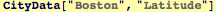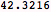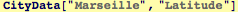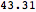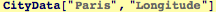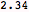Introduce a function that finds the spherical coordinates of a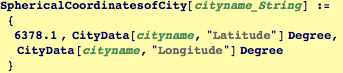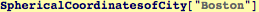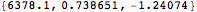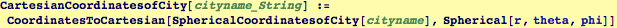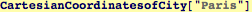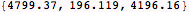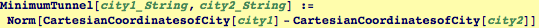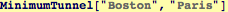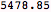Minimum travel distance between Boston and Paris for a round earth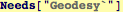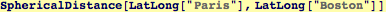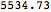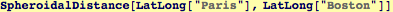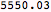Created by Wolfram Mathematica 6.0  (21 September 2007)